Using AMPL Studio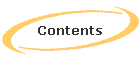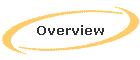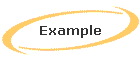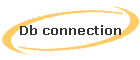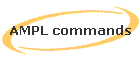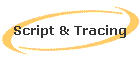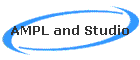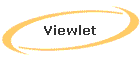# Introducing AMPL through AMPL Studio

## Introduction to Models for Linear programming

In order to suitably represent the linear programs we make use of mathematical notations. We call the compact description of the general form of the problem, as a ‘model’. The fundamental components of a model are:

·        Sets

·        Parameters

·        Variables, whose values the solver is to determine

·        An Objective, to be maximized or minimized

·        Constraints, that the solution must satisfy

The example below shows a symbolic model:

Given:      P, a set of products

aj = Tons per hour of product j, for each j Є P

b = hours available at the mill

cj = profit per ton of product j, for each j Є P

uj = maximum tons of product j, for each j Є P

Define variables: Xj = tons of product to be made, for each j Є P

Maximize: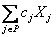Subject to:Figure 5.1: A symbolic production model in algebraic form

## Fundamental Components of AMPL linear programming Model

### Sets

Unordered Sets

The most elementary kind of AMPL set is an unordered collection of character strings. Usually all of the strings in a set are intended to represent instances of the same kind of entity.

The declaration of a set need only contain the keyword ‘set’ and a name. For example a model may declare

set PROD;

to indicate that a certain set will be referred to by the name PROD in the rest of the model. A name may be any sequence of letters, numerals, and underscore (_) characters that is not a legal number. A few names have special meanings in

AMPL and may only be used for specific purposes, while a large number of names have predefined names that can be changed if they are used in some other way.

A declared set’s membership is normally specified as part of the data for the model. Occasionally, however, it is desirable to refer to a particular set of strings within a model. A literal set of this kind is specified by listing its members within braces:

set PROD = {“bands”, “coils”, “plate”};

This sort of declaration is best limited to cases where a set’s membership is small, is a fundamental aspect of the model, or is not expected to change often.

Sets of numbers

Set members may also be numbers. In fact a set’s members may be mixture of numbers and strings, though this is seldom the case. In an AMPL model, a literal number is written in the customary way as a sequence of digits, optionally preceded by a sign, containing an optional decimal point, and optionally followed by an exponent; the exponent consists of a d, D, e or E, optionally a sign, and a sequence of digits.

A set of numbers is often a sequence that corresponds to some progression in the situation being modeled, such as a series of weeks or years. Just as for strings, the numbers in a set can be specified as part of the data, or can be specified within a model as a list between braces, such as {1, 2, 3, 4, 5, 6}. This sort of set can be described more concisely by notation 1..6. An addition ‘by’ clause can be used to specify an interval more than 1 between the numbers; for instance,

1990.. 2020 by   5

Represents the set

{1990, 1995, 2000, 2005, 2010, 2015, 2020}

This kind of expression can be used anywhere that a set is appropriate.

The members of a set of numbers have the same properties as any other numbers, and hence can be used in arithmetic expressions.

Set Operations

AMPL has four operators that construct new sets from existing ones:

A union B;         #union: in either A or B

A inter B;         #intersection: in both A and B

A diff B;          #difference: in A but not B

A symdiff B;       #symmetric difference: in A or B but not both

The following example shows how this work:

ampl:set Y1 = 1990 .. 2020 by 5;

ampl:set Y2 = 2000 .. 2025 by 5;

ampl: display Y1 union Y2, Y1 inter Y2;

set Y1 union Y2 := 1990 1995  2000  2005  2010  2015  2020  2025;

set Y1 inter Y2 := 2000 2005  2010  2015  2020;

ampl: display Y1 diff Y2, Y1 symdiff Y2;

set Y1 diff Y2 := 1990  1995;

set Y1 symdiff Y2 := 1990 1995 2025;

Set membership operations and functions

Two other AMPL operators, ‘in’ and ‘within’, test the membership of sets. As an example the expression

“B2” in NUTR

Is true if and only if the string “B2” is a member of the set NUTR. The expression

MINREQ within NUTR

is true if all members of the set MINREQ are also members of NUTR – that is, if MINREQ is a subset of(or is same as) NUTR.

AMPL also provides ‘not in’ and ‘not within’, which reverses the truth value of their results.

he built in function ‘card’ computes the number of members in (or cardinality of) a set; for example, card(NUTR),is the number of the members in NUTR.

Indexing Expressions

In algebraic notation, the use of sets is indicated informally by phrases such as “for all i Є P” or “for t=1,…,T” or “for all j Є R such that cj > 0.” The AMPL counterpart is the indexing expression that appears within braces { … }. An indexing expression is used whenever we specify the set over which a model component is indexed, or the set over which a summation runs. Since an indexing expression defines a set, it can be used in any place where a set is appropriate.

The simplest form of indexing expression is just a set name or expression within braces. For example:

param rate {PROD} > 0 ;

param avail {1..T} > = 0;

References to these parameters are subscripted with a single set member, in expression such as avail[t] and rate[p].

The names such as t or i that appear in subscripts and other expressions in our models are examples of dummy indices that have been defined by indexing expressions. In fact, any indexing expression may optionally define a dummy index that runs over the specified set.

An indexing expression consists of an index name, the keyword ‘in’, and a set expression as before.  Although a name defined by a model component’s declaration is known throughout all subsequent statements in the model, the definition of dummy index name is effective only within the scope of the defining indexing expression. Once an indexing expression’s scope has ended, its dummy index becomes undefined. Thus the same index name can be defined again and again in the model.

As a final option, the set in an indexing expression may be followed by a colon(:) and a logical condition. The indexing expression then represents only the subset of members that satisfy the condition. For example:

{j in FOOD: f_max [j] – f_min[j] < 1}

describes the set of all foods whose minimum and maximum amounts are nearly the same.

Ordered Sets

Any set of numbers has a natural ordering, so numbers are often used to represent entities, like time periods, whose ordering is essential to the specification of a model. To describe the difference between this week’s inventory and the previous week’s inventory, for example, we need the weeks to be ordered so that the “previous” week is always well defined.

An AMPL model can also define its own ordering for any set of numbers or strings, by adding the keyword ‘ordered’ or ‘circular’ to the set’s declaration. The order in which we give the set’s members, in either the model or data, is the order in which AMPL works with them. In a set declared ‘circular’, the first member is considered to follow the last one, and the last to precede the first; in an ordered set, the first member has no predecessor and the last member has no successor.

There are many functions on ordered sets to retrieve some specific members from the set. Users are referred to AMPL manual or AMPL textbook for further details.

### Parameters

In AMPL a single named numerical value is called parameter. Although some parameters are defined as individual scalar values, most occur in vectors or matrices or other collections of numerical values indexed over sets. Parameters and other numerical values are the building blocks of the expressions that make up a model’s objective and constraints.

Parameter declarations have a list of optional attributes, optionally separated by commas:

parameter declaration:

param name aliasopt indexingopt attributesopt ;

The attributes may be any of the following:

attribute:

binary

integer

symbolic

relop expr

In sexpr

= expr

Default expr

relop:

< <= = == != <> > >=

The keyword integer restricts the parameter to be an integer; binary restricts it to 0 or 1. If symbolic is specified, then the parameter may assume any literal or numeric value, and the attributes involving <.<=,>= and > are disallowed; otherwise the parameter is numeric and can only assume a numeric value.

The attributes involving comparison operators specify that the parameter must obey the given relation. The = and default attributes are analogous to the corresponding ones in set declarations and are mutually exclusive.

Recursive definitions of indexed parameters are allowed, so long as the assigned values can be computed in a sequence that only references previously computed values. For example:

param comb ‘n choose k’ {n in 0..N, k in 0..n}

= if k = 0 or k = n then 1 else comb [n-1,k-1] + comb[n-1,k];

Computes the number of ways of choosing n things k at a time.

### Variables

The variables of a linear program have much in common with its numerical parameters. Both are symbols that stand for numbers, and that may be used in arithmetic expressions. Parameter values are supplied by the modeler or computed from other values, while the values of variables are determined by an optimizing algorithm. Syntactically, variable declarations are the same as the parameter declaration defined earlier, except that they begin with the keyword ‘var’ rather than ‘param’. The meaning of qualifying phrases within the declaration may be different, however when these phrases are applied to variables rather than to parameters.

Phrases beginning with >= or <= are by far the most common in declarations of variables for linear programs. For example:

var Make {p in PROD} >=0, <= market[p];

The declaration creates an indexed collection of variables Make[p], one for each member p of the set PROD; the rules in this respect are exactly the same as for parameters. The effect of the two qualifying phrases is to impose a restriction, or constraint, on the permissible values of the variables. Specifically, >= 0 implies that all of the variables Make[p] must be assigned non negative values by the optimizing algorithm, while the phrase <=market[p]says that, for each product p, the value given to Make[p] may not exceed the value of the parameter market[p].In general, either >= or <= may be followed by an arithmetic expression in previously defined sets and parameters and currently defined dummy indices. The values following >= and <= are lower and upper bounds on the variables.

An = phrase in a variable declaration gives rise to a definition, as in parameter declaration. Because a variable is being declared, however, the expression to the right of = operator may contain previously declared variables as well as sets and parameters.

A := or ‘default’ phrase in a variable declaration gives initial values to the indicated variables. Variables are not assigned an initial value by := can also be assigned initial values from a data file.

Finally, variables can be defined as ‘integer’ or ‘binary’.

Linear Expressions

An arithmetic expression is linear in a given variable if, for every unit increase or decrease in the variable, the value of expression increases or decreases by some fixed amount. An expression that is linear in all its variables, is called a linear expression.

AMPL recognizes as a linear expression any sum of terms of the form:

constant-expr

variable-ref

(constant-expr) * variable ref

Provided that each constant-expr is an arithmetic expression that contains no variables, while var-ref is a reference to a variable. The parentheses around the constant-expr may be omitted if the result is the same according to the rules of operator precedence.

### Objectives

The declaration of an objective function consist of one of the keywords minimize or maximize, a name, a colon, and a linear expression in previously defined sets, parameters and variables. For example:

minimize Total_cost: sum {j in FOOD} cost[j] * Buy[j];

and

maximize Total_Profit:

sum {p in PROD, t in 1..T}

(sum {a in AREA[p] revenue[p,a,t] * Sell[p,a,t] – prodcost[p] * Make[p,t] – invcost[p] * Inv[p,t]);

Within AMPL commands, the objective’s name refers to its value.

Although a particular linear program must have one objective function, a model may contain more than one objective declaration. Moreover, any minimize or maximize declaration may define an indexed collection of objective functions, by including an indexing expression after the objective name. In these cases, we may issue an objective command, before typing solve, to indicate which objective is to be optimized.

### Constraints

The simplest kinds of constraint declaration begins with the keywords subject to, a name, and a colon. Even the subject to is optional; AMPL assumes that any declaration not beginning with a keyword is a constraint. Following the colon is an algebraic description of the constraint, in terms of previously defined sets, parameters and variables. For example:

subject to  Time:

sum{p in PROD} (1/rate[p])* Make[p] <= avail;

The name of a constraint, like the name of an objective, is not used anywhere else in an algebraic model, though it figures in alternative “columnwise” formulations and is used in AMPL command environment to specify the constraint’s dual value and other associated quantities.

Most of the constraints in large linear programming models are defined as indexed collections, by giving an indexing expression after the constraint name.

The constraint Time, for example, is generalized in the subsequent example to say that the production time may not exceed the time available in each processing stage s.

subject to Time{s in STAGE}:

sum {p in PROD} (1/rate[p,s])* Make[p] <= avail[s];

The indexing expression in a constraint declaration should specify a dummy index for each dimension of the indexing set.

AMPL’s algebraic description of a constraint may consist of any two linear expressions separated by an equality or inequality operator:

linear-expr <= linear-expr

linear-expr = linear-expr

linear-expr >= linear-expr

While it is customary in mathematical descriptions of linear programming to place all terms containing variables to the left of the operator and all other terms to the right, AMPL imposes no such requirement. AMPL also allows double inequality constraints. The permissible forms for a constraint of this kind are:

const-expr <= linear-expr <= const-expr

const-expr <= linear-expr <= const-expr

where each const-expr must contain no variables. The effect is to give upper and lower bounds on the value of the linear-expr.

The example below gives the AMPL model and data files for the symbolic algebraic model considered in the beginning of this chapter.

set P;

param a {j in P};

param b;

param c {j in P};

param u {j in P};

var x {j in P};

maximize Total_Profit: sum {j in P} c[j]* X[j];

subject to Time: sum {j in P} (1/a[j]) * X[j] <= b;

subject to Limit {j in P}: 0 <= X[j] <= u[j] ;

Figure 5.2: Basic production model in AMPL

set P := bands coils;

param:      a     c     u     :=

bands      200   25    6000

coils      140   30    4000  ;

param b := 40;

Figure 5.2: Production model data file in AMPL

## Stochastic Extension to AMPL: SAMPL

In addition to supporting AMPL language syntax for deterministic problems, AMPL Studio has an extension for stochastic programming called SAMPL, available as a separate package.

SAMPL has additional syntax and commands. Users are referred to SAMPL manual for more details.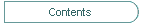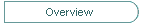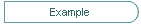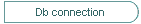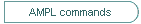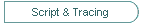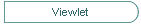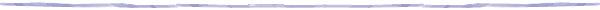Copyright (c) 2012. Datumatic Ltd. Registration No. 04988675. UK.